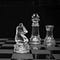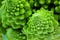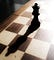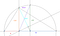# The Knight’s Tour: Where Chess, Programming, and Math MeetPhoto by Wim van 't Einde on Unsplash

Besides bringing a beautiful game, chess also brought interesting math and algorithms. In this article, we will explore one of the most famous and mysterious problems: The Knight’s Tour. First, we will explore some proofs and then we will code the backtracking algorithm that finds solutions.

# Mathematics behind the problem

The knight is a curious piece in chess, as it has the “L-move” in any direction. The Knight’s Tour is a problem that asks if the knight can go through all of the 64 squares of a chess board without landing in the same square twice. …

# Towers of Hanoi in Python: Recursive Algorithm and Animation

The Tower of Hanoi is an old puzzle. Yet, it continues to amaze us with its elegant solution, which is hidden by its simplicity.

The animations shown in this article are constructed on a python library called Manim. This particular animations were based on the ones used in a YouTube Video by Reducible.

First we need to understand the small cases. Then, we are going to generalize for more disks. Finally, we will build a recursive algorithm that solves it in the least steps possible. Animations will help along the way.

# Vocabulary and Notation

• Rod: Things where the disks can be placed. There…

# 7 Ways to Code the Fibonacci Algorithm in PythonPhoto by Paul Milasan on Unsplash

In this article we are going to use this problem to explain and compare some algorithms that are different but can achieve the same task. To view the difference between them, each one will calculate the same Fibonacci number, the 50th one (if possible). As it is Python, the index will start from 0. So, the 0th term is 1, the 1st term is 1, the 2nd term is 2, and so on.

Time will be measured in each one, and then they will be compared. For this we need the time package.

`import time`

# 1. Generate Series

This is the most simple…

# Solve the 8 Queens Problem in PythonPhoto by Alexis Fauvet on Unsplash

Before starting, let me clarify that this solution is neither the best of time complexity nor the most efficient. However, I found this solution to be easy to understand and code, and it solves them in a quick time.

There are some algorithms that use Backtracking or Branch and Bound, which require understanding in specific algorithms. Our approach is based on brute force, but intelligent brute force with a module called Itertools. First, let’s talk about some math.

# Levels of Brute Force

Our objective is to go through the least possible arrangements to check the conditions. …

# Minimize Sum: Geometry Problem with a clever solution

The problem goes as follows. “Given a rectangle ABCD where AB=1 and BC=2, P, Q are on BD, BC respectively. Find the smallest possible value of CP+PQ.”

Give it a try before you continue reading. If you are already familiar with this type of problems, you might find the solution quickly. However, if you have no idea how to solve them, you will be impressed by the elegance of it!

# Key Thought

Suppose we fix P. Which is the best Q we can choose? There is a theorem that might help us with that.

The least distance between a point and a…

# Mean Inequalities are the opposite of mean and unequalGeometric representation of the means with values a and b.

Inequalities are often seen as not useful, except for mathematical competitions. However, they do have some applications and the secrets that they hide are surprising.

# Introduction to Means

A mean is a mathematical or statistical operation where you find an average value between two or more quantities. The resulting value lies between the smallest and the biggest numbers.

A weighted mean is a type of mean used when each value has different importance or weight. For example, schools use this to obtain the GPA as the exam has a higher weight than a homework. …